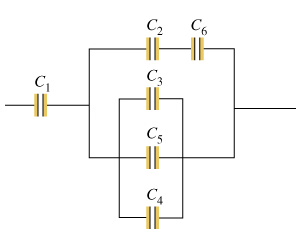# Problem: Consider the combination of capacitors shown in the diagram, where C1 = 3.00 μF , C2 = 11.0 μF , C3 = 3.00 μF , and C4 = 5.00 μF . (Figure 1)Two capacitors of capacitance C5 = 6.00 μF and C6 = 3.00 μF are added to the network, as shown in the diagram (Figure 2) Find the equivalent capacitance CB of the new network of capacitors. Express your answer in microfarads.

###### FREE Expert Solution

C3, C5 and C4 are in parallel:

C3,5,4 = (C3 + C5 + C4) = (3.00 + 6.00 + 5.0) = 14.0  μF

99% (79 ratings)###### Problem Details

Consider the combination of capacitors shown in the diagram, where C1 = 3.00 μF , C2 = 11.0 μF , C3 = 3.00 μF , and C4 = 5.00 μF . (Figure 1)Two capacitors of capacitance C5 = 6.00 μF and C6 = 3.00 μF are added to the network, as shown in the diagram (Figure 2)